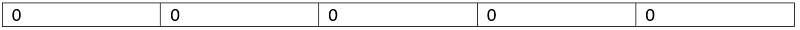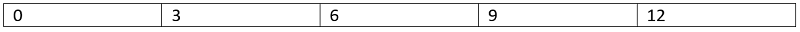Q:

# Knapsack with Duplicate Items

Weights and values are given for n items along with the maximum capacity allowed W. What is the maximum value we can achieve if we can pick any weights, any number of times for the total allowed capacity of W?

Input:

First line contains two integers N and W denoting the number of items and the total allowed weight.

In the next two lines are space separated values of the array denoting values of the items val[n] and their weights weights[n] respectively.

Output:

Output the maximum value which we can achieve if we can pick any weights any number of times for a total weight capacity of W.

Constraints:

```1 <= N,W <= 100
1 <= weight[i], val[i] <= 100
```

Example:

```Test case: 1

Input:
N=2,W= 3
val[]=1 1
weight[]=2 1

Output:
Maximum value that can be achieved: 3

Test case: 2

Input:
N=3,W= 4
val[]=2 5 3
weight[]=1 2 1

Output:
Maximum value that can be achieved: 12
```

Explanation:

```For the first test case,
Will use the second item with weight 1 only.
We use will 3 such item to have maximum value 3.
Any other combinations will give less value

For the second test case,
Some possible combinations can be
Writing as (value, weight) pair
(2,1), (2,1), (2,1), (2,1)- total weight 4, value 8
(2,1), (2,1), (5,2) - total weight 4, value 9
...
(3,1),(3,1), (3,1),(3,1)- total weight 4, value 12

The last combination is the most optimal one
and that's produces the maximum.```

The difference between this problem and the general knapsack problem is here we can use the same item several times. So the simple recursion about either choosing an item or leaving the item will not work here as using the same item may lead to the most optimized solution.

Hence, the algorithm will be like following,

1. Initialize a dp[w+1] to store for each sub-problem up to total capacity W.
2. Reset all the values to zero initially.
memset(dp,0,sizeof(dp));
3. Fill up the DP table with base value dp=0,
```for sub-problem weight,i=1 to w
for a weight,wj in the weight array
if i>=wj
dp[i] = std∷max⁡(dp[i],dp[i-wj]+valj)
end if
end for
end for
```
4. Maximum value that can be achieved is dp[w]

Let's compute the above for our test case two,

```N = 3, W = 4
val[] = 2 5 3
weight[] = 1 2 1
```

Initially the DP table is,To compute dp,

```weight<=1

So,
dp = max(dp,dp[1-1]+val) = 2

Weight>1. So it can't have any role while computing DP
Weight<=1

So,
dp = max(dp,dp[1-1]+val) = 3
```

Similar way you can hand compute the DP table to understand how the algo is performing is reaching to the optimal answer.

The final DP table for the above test case would look like,Where 12 is the final case.

C++ Implementation:

``````#include <bits/stdc++.h>
using namespace std;

void print(vector<int> a, int n)
{
for (int i = 0; i < n; i++)
cout << a[i] << " ";
cout << endl;
}

int my(vector<int> price, vector<int> weight, int n, int w)
{

int dp[w + 1];
memset(dp, 0, sizeof(dp));

for (int i = 1; i <= w; i++) {
for (int j = 1; j <= n; j++) {
if (i >= weight[j - 1])
dp[i] = std::max(dp[i], dp[i - weight[j - 1]] + price[j - 1]);
}
}

return dp[w];
}

int main()
{
int n, item, w;

cout << "enter number of item and total weights\n";
cin >> n >> w;

cout << "Enter the prices\n";
vector<int> price;

for (int j = 0; j < n; j++) {
scanf("%d", &item);
price.push_back(item);
}

cout << "Enter the weights\n";
vector<int> weight;

for (int j = 0; j < n; j++) {
scanf("%d", &item);
weight.push_back(item);
}

cout << "result is: " << my(price, weight, n, w) << endl;

return 0;
}``````

Output:

```enter number of item and total weights
3 4
Enter the prices
2 5 3
Enter the weights
1 2 1
result is: 12```